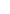## The 555 timer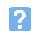1. The simplified internal arrangement of a 555 timer is shown in the diagram below. The function of the pin marked X is: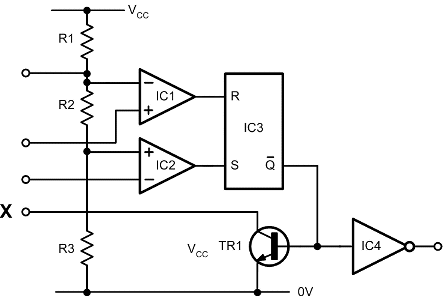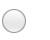a) Controlb) Dischargec) Thresholdd) Trigger.2. What time period is is generated by the circuit shown below?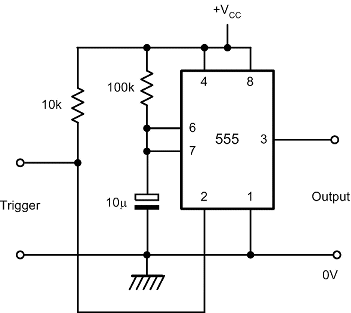a) 0.11 secondsb) 0.37 secondsc) 0.63 secondsd) 1.1 seconds.3. In the circuit shown, the trigger input must be:a) taken low to end the timing periodb) taken high to end the timing periodc) taken low to start the timing periodd) taken high to start the timing period.4. In the circuit shown below, the low time of the waveform generated by the timer will depend on: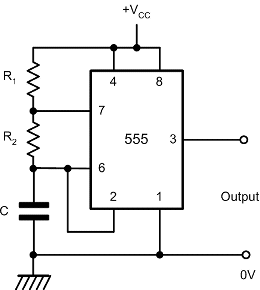a) C and R1 onlyb) C and R2 onlyc) R1 and R2 onlyd) C and (R1 + R2).5. In order to produce a square wave with a near 50% duty cycle from the circuit shown in the previous question, the component values should be chosen so as to satisfy the relationship:a) R1 = R2b) R1 << R2c) R2 << R1d) C x R1 = C x R2.6. Which one of the following gives the period of the waveform shown in the diagram below: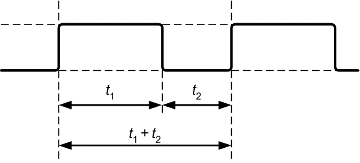a) t1b) t2c) t1/t2d) t1 + t2.7. Which one of the following gives the duty cycle of the waveform shown?a) t1/t2 x 100%b) t2/t1 x 100%c) t1/(t1+t2) x 100%d) (t1 - t2)/(t1 + t2) x 100%.8. What packge is used for this 555 timer?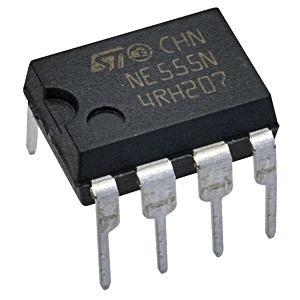a) Dual 4-pinb) 8-pin surface mountc) 8-pin dual-in-lined) 8-pin flatpack.
This is more feedback!
This is the feedback!